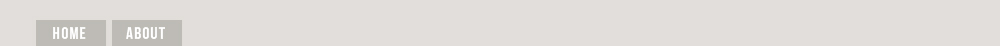## Feb 28, 2021

### Computers Are Inventing Entirely New Math Humans Have Never Seen Before

This is intended to assist mathematicians, not replace them. But then we've heard that before. JL

Mordechai Rorvig reports in Motherboard:

An automated conjecturing system is named after the mathematician Srinivasa Ramanujan, who developed thousands of innovative formulas in number theory with no formal training. The software system has conjectured several original and important formulas for universal constants that show up in mathematics. This appears to mark a new progress point for computing, somewhat like the first time that computers beat out the chessmasters; but this time, in the game of making conjectures.

A good conjecture has something like a magnetic pull for the mind of a mathematician. At its best, a mathematical conjecture states something extremely profound in an extremely precise and succinct way, crying out for proof or disproof.

But posing a good conjecture is difficult. It must be deep enough to provoke curiosity and investigation, but not so obscure as to be impossible to glimpse in the first place. Many of the most famous problems in mathematics are conjectures, and not solutions, such as Fermat’s last theorem.

Now, a group of researchers from the Technion in Israel and Google in Tel Aviv presented an automated conjecturing system that they call the Ramanujan Machine, named after the mathematician Srinivasa Ramanujan, who developed thousands of innovative formulas in number theory with almost no formal training. The software system has already conjectured several original and important formulas for universal constants that show up in mathematics. The work was published last week in Nature

One of the formulas created by the Machine can be used to compute the value of a universal constant called Catalan’s number more efficiently than any previous human-discovered formulas. But the Ramanujan Machine is imagined not to take over mathematics, so much as provide a sort of feeding line for existing mathematicians.

As the researchers explain in the paper, the entire discipline of mathematics can be broken down into two processes, crudely speaking: conjecturing things and proving things. Given more conjectures, there is more grist for the mill of the mathematical mind, more for mathematicians to prove and explain.

That’s not to say their system is unambitious. As the researchers put it, the Ramanujan Machine is “trying to replace the mathematical intuition of great mathematicians and providing leads to further mathematical research.”

The researchers’ system is not, however, a universal mathematics machine. Rather, it conjectures formulas for how to compute the value of specific numbers called universal constants. The most famous of such constants, pi, gives the ratio between a circle’s circumference and diameter. Pi can be called universal because it shows up all across mathematics, and constant because it maintains the same value for every circle, no matter the size.

In particular, the researchers’ system produces conjectures for the value of universal constants (like pi), written in terms of elegant formulas called continued fractions. Continued fractions are essentially fractions, but more dizzying. The denominator in a continued fraction includes a sum of two terms, the second of which is itself a fraction, whose denominator itself contains a fraction, and so on, out to infinity.

Continued fractions have long compelled mathematicians with their peculiar combination of simplicity and profundity, with the total value of the fraction often equalling important constants. In addition to being “intrinsically fascinating” for their aesthetics, they are also useful for determining the fundamental properties of the constants, as Robert Doughtery-Bliss and Doron Zeilberger of Rutgers University wrote in a preprint from 2020.

The Ramanujan Machine is built off of two primary algorithms. These find continued fraction expressions that, with a high degree of confidence, seem to equal universal constants. That confidence is important, as otherwise, the conjectures would be easily discarded and provide little value.

Each conjecture takes the form of an equation. The idea is that the quantity on the left side of the equals sign, a formula involving a universal constant, should be equal to the quantity on the right, a continued fraction.

To get to these conjectures, the algorithm picks arbitrary universal constants for the left side and arbitrary continued fractions for the right, and then computes each side separately to a certain precision. If the two sides appear to align, the quantities are calculated to higher precision to make sure their alignment is not a coincidence of imprecision. Critically, formulas already exist to compute the value of universal constants like pi to an arbitrary precision, so that the only obstacle to verifying the sides match is computing time.

Prior to algorithms such as this, mathematicians would have needed to use existing mathematical knowledge and theorems to make such a conjecture. But with the automated conjectures, mathematicians may be able to use them to reverse engineer hidden theorems or more elegant results, as Doughtery-Bliss and Zeilberger have already shown.

But the researchers’ most notable discovery so far is not hidden knowledge, but a new conjecture of surprising importance. This conjecture allows for the computation of Catalan’s constant, a specialized universal constant whose value is needed for many mathematical problems.

The continued fraction expression of the newly discovered conjecture allows for the most rapid computation yet of Catalan’s constant, beating out prior formulas, which took longer to crank through the computer. This appears to mark a new progress point for computing, somewhat like the first time that computers beat out the chessmasters; but this time, in the game of making conjectures.